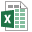# TeamDynamix Project Request Scoring

## Overview

It is common for institutions to score project requests in order to determine the value of the request. Project requests are generally an “Apples-to-Oranges” comparison, making it difficult to decide between requests. Scoring aims to eliminate some of the differences so that requests can be compared more accurately. It is common in the industry to score projects on a 100 point scale. Within TeamDynamix, the Goals, Risks, and Scorecard/Criteria sections make-up the total score. Configuring these sections is described in the previous section. The following describes how each section contributes to the overall score. Additionally, a spreadsheet is attached to this article which includes a mocked, up score card example to highlight how the score is calculated.

## Goals Calculation

The score contributed to the composite score is a function of the number of goals supported by the project (which produces a number between 0-1) multiplied by the Goals PCT of Value multiplier. The max goal score is 1 if project is aligned with all goals. The Goals contribution to the composite score will be calculated by multiplying the goals score (again, a value between 0-1) by the % value multiplier to the score. The equation to calculate the goals score is as follows:

Goals’ contribution to the composite score = ((Number of Goals Supported/Total Number of Goals)* % value multiplier)

So as an example, if there are 4 total goals from which to select and a project aligns with 3 of the 4 the goals score will be .75. Furthermore, if the % contribution for goals is set to 3000% in the TeamDynamix Administration tool, the calculation becomes 0.75 * 3000% = 22.5 out of a possible 30 points.

## Risks Calculation

The weight added to the composite score by the project risks is a function of the summed value of all of the risks (between 0 and 1) and the Risks PCT of Value Multiplier. Each risk entered in the system has choices of NA, Low, Medium and High. If there is high risk, 0 points are given and if there is no risk (N/A), 1 point is given. The scoring values of each choice are illustrated below:

N/A = 1
Low = .67
Medium = .33
High = 0

The equation to calculate the risk score is as follows:

(Sum of All Selected Choice Weights for All Risks)/(Total Number of Risks)* % Value Multiplier

So as an example, if there are 5 risks in total and 3 are N/A (each equal to 1 point), 1 risk is High (=0), and 1 risk is Medium (=.33) the un-weighted risk score would be 3.33. Furthermore, if the % contribution for goals is set to 3500% in the TeamDynamix Administration tool, the calculation becomes (3.33/5) * 3500% = 23.31 out of a possible 35 total points.

## Scorecard Criteria Calculation

The weight added to the scorecard and composite score by an individual scorecard criterion is a function of the value of the criterion (between 0 and 1) and the % PCT of Value Multiplier. The value of the criterion is multiplied by the % Value to determine the total value contributed to the score. The equation is as follows:

Value Contributed to the Score = (Selected Choice Value/Max Choice Value)* % Score Multiplier

As an example, assume a criterion with a % value of 600% and 5 choices with the following values:

Choice N/A = 0
Choice A = 1
Choice B = 2
Choice C = 3
Choice D = 4

If N/A is selected then the value contributed to the scorecard is ((0/4) * 600%) = 0
If A is selected then the value contributed to the scorecard is ((1/4) * 600%) = 1.5
If B is selected then the value contributed to the scorecard is ((2/4) * 600%) = 3
If C is selected then the value contributed to the scorecard is ((3/4) * 600%) = 4.5
If D is selected then the value contributed to the scorecard is ((4/4) * 600%) = 6

## Composite Score PCT Value

The Composite Score PCT Value represents the relative position of the request in relation to the total possible score at the time it was scored. For example, if there is a total possible score of 100 points and the project has a score of 50 then the composite score % will be 50%. If at some point in the future the scoring is changed so that the max score is 120 the composite score % helps to clarify that when the project request was originally scored it achieved 50% of the possible points even though with today's model (120 total possible) it would be 42%. It allows for changes to the scoring model yet still evaluate the relative score of projects scored under a different model.

## 10.2 brought multiple scorecard functionality

Now, you can create mutliple scorecards and decide which scorecard criteria are then associated with each scorecard. This is also configured within admin.

### Details

Article ID: 12511
Created
Sun 4/24/16 10:49 PM
Modified
Wed 7/10/19 9:18 PM

### Files (1)#### TeamDynamix Scorecard Calculation.xlsx

4/30/2016 3:06:41 PM